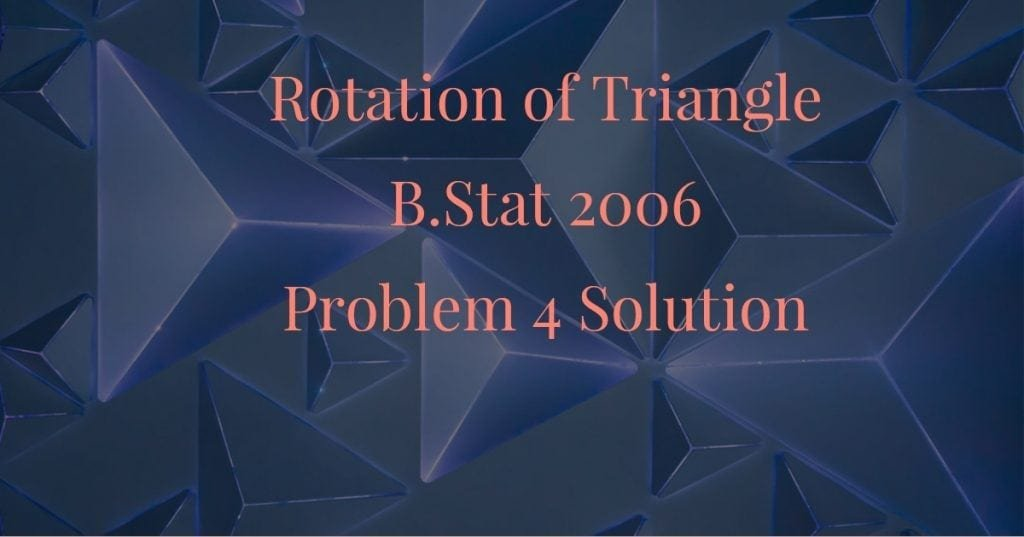How Cheenta works to ensure student success?
Explore the Back-Story

# Rotation of triangle (B.Stat 2006, Problem 4 solution)Problem:

In the figure below, $E$ is the midpoint of the arc $ABEC$ and the segment $ED$ is perpendicular to the chord $BC$ at $D$. If the length of the chord $AB$ is $\mathbf{\ell_1}$, and that of the segment $BD$ is $\mathbf{\ell_2}$, determine the length of $DC$ in terms of $\mathbf{\ell_1, \ell_2}$.

Discussion:

Teacher: Here is a clue: rotate ($\Delta EDC$ ) about point $E$ such that $EC$ falls along $EA$. Can you draw the diagram after rotation?

Student:

Obviously $EC$ will fit into $EA$ as $E$ is the midpoint of larger arc $AC$. Suppose $D$ falls on $D'$. Then $ED'A$ is the rotated form of $EDC$.

Teacher: Can you show that $D'A$ and $BA$ are the same line?

Student: Okay I can try. If we can show that $\angle D'AE = \angle BAE$ , then we have shown that $D'A$ and $BA$ is the same line. Now $\angle D'AE = \angle DCE = \angle BCE$ (due to rotation) . But $\angle BCE = \angle BAE$ as they are the angle subtended by the same segment $BE$.

So we have $\angle D'AE = \angle BAE$. Therefore $D'A$ falls on $BA$.

Teacher: Let's revise the diagram then.

Now can you finish the problem?

Student: Since $\Delta E_1 D_1' A_1 = \Delta E_1 D_1 C_1$ due to rotation, we have $E_1 D_1' = E_1 D_1 \Rightarrow \angle E_1 D_1' D_1 = \angle E_1 D_1 D_1'$

But $\angle E_1 D_1' B_1 = \angle E_1 D_1 B_1' = 90^{\circ} \\ \Rightarrow \angle E_1 D_1' B_1 - \angle E_1 D_1' D_1 \\ = \angle E_1 D_1 B_1' - \angle E_1 D_1 D_1' \Rightarrow \angle B_1 D_1' D_1 \\ = \angle B_1 D_1 D_1' \Rightarrow B_1 D_1 \\ = B_1 D_1 '$

Thus $C_1D_1 = A_1 D_1' \\ = A_1 B_1 + B_1 D_1' \\= A_1 B_1 + B_1 D_1 \\= \ell_1 + \ell_2$

Problem:

In the figure below, $E$ is the midpoint of the arc $ABEC$ and the segment $ED$ is perpendicular to the chord $BC$ at $D$. If the length of the chord $AB$ is $\mathbf{\ell_1}$, and that of the segment $BD$ is $\mathbf{\ell_2}$, determine the length of $DC$ in terms of $\mathbf{\ell_1, \ell_2}$.

Discussion:

Teacher: Here is a clue: rotate ($\Delta EDC$ ) about point $E$ such that $EC$ falls along $EA$. Can you draw the diagram after rotation?

Student:

Obviously $EC$ will fit into $EA$ as $E$ is the midpoint of larger arc $AC$. Suppose $D$ falls on $D'$. Then $ED'A$ is the rotated form of $EDC$.

Teacher: Can you show that $D'A$ and $BA$ are the same line?

Student: Okay I can try. If we can show that $\angle D'AE = \angle BAE$ , then we have shown that $D'A$ and $BA$ is the same line. Now $\angle D'AE = \angle DCE = \angle BCE$ (due to rotation) . But $\angle BCE = \angle BAE$ as they are the angle subtended by the same segment $BE$.

So we have $\angle D'AE = \angle BAE$. Therefore $D'A$ falls on $BA$.

Teacher: Let's revise the diagram then.

Now can you finish the problem?

Student: Since $\Delta E_1 D_1' A_1 = \Delta E_1 D_1 C_1$ due to rotation, we have $E_1 D_1' = E_1 D_1 \Rightarrow \angle E_1 D_1' D_1 = \angle E_1 D_1 D_1'$

But $\angle E_1 D_1' B_1 = \angle E_1 D_1 B_1' = 90^{\circ} \\ \Rightarrow \angle E_1 D_1' B_1 - \angle E_1 D_1' D_1 \\ = \angle E_1 D_1 B_1' - \angle E_1 D_1 D_1' \Rightarrow \angle B_1 D_1' D_1 \\ = \angle B_1 D_1 D_1' \Rightarrow B_1 D_1 \\ = B_1 D_1 '$

Thus $C_1D_1 = A_1 D_1' \\ = A_1 B_1 + B_1 D_1' \\= A_1 B_1 + B_1 D_1 \\= \ell_1 + \ell_2$

This site uses Akismet to reduce spam. Learn how your comment data is processed.

### One comment on “Rotation of triangle (B.Stat 2006, Problem 4 solution)”

1. […] Rotation of triangle (B.Stat 2006, Problem 4 solution) […]

### Knowledge Partner